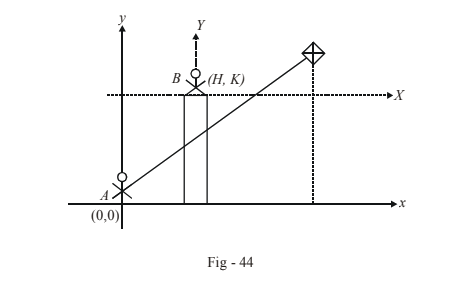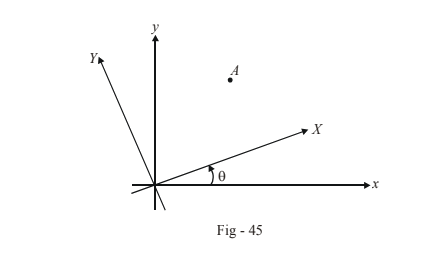# Transformation of Coordinates

Go back to  'Straight Lines'

Suppose that a person A is flying a kite from the ground while another person B is observing this kite from the top of a building, as shown below:In A's frame of reference, the coordinates of B are (H , K).  Now suppose that A and B both specify the position of the kite relative to themselves. It should be evident that the coordinates of the kite in the two reference frames will be different.

Let the coordinates of the kite be (x ,y )  in A's reference frame and (X ,Y )  in B's reference frame. Then, we have

$X = x - H\;\;,\;\;\;\;\;\;\;\;\;\;\;\;Y = y - K \qquad\quad\cdots (1)$

Thus, a translation of the axes implies a corresponding change in the coordinates in the manner specified by (1). In fact, if the kite traces a path $$f(x,y) = 0$$ in A's reference frame, it will trace the path $$f(X + H,Y + K) = 0$$ in B's frame of reference.

Translation of axes implies a simple shift in the origin without a change in the relative orientation of the axes. We now consider the case when the axes is rotated but the origin is the sameLet a point A have the coordinates ( x, y) in the original frame of reference and (X , Y)  in the rotated frame of reference. Verify that the following relations hold true :

$x = X{\rm{cos}}\theta - {\rm{Ysin}}\theta$

$y = X\sin \theta + Y\cos \theta$

We can now combine the case of translation and rotation of axes to determine the most general transformed coordinates. Let the origin of the axes be shifted to (h , k)  and the axes be rotated by an anticlockwise angle $$\theta$$.

The original coordinates (x , y)  and the coordinates in the new frame of reference (X , Y) will satisfy the relations

\begin{align}x &= h + X\cos \theta - Y\sin \theta \\y &= k + \sin \theta + Y\cos \theta \end{align}